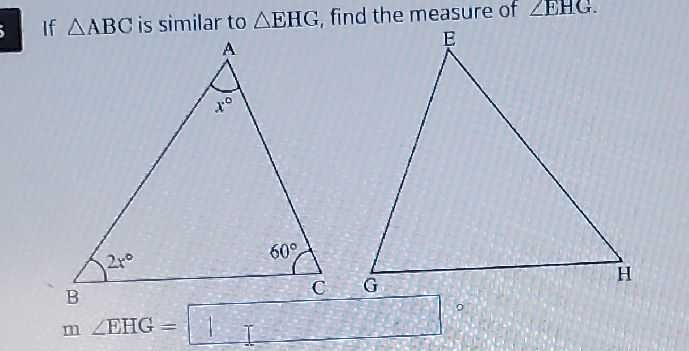### Still have math questions?If $$\triangle ABC$$ is similar to $$\triangle EHG$$ , find the measure of $$\angle EHC$$ .